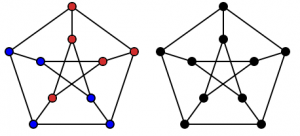Syamand Hasam, The University of Sydney

One of the interesting parts of my research this summer was trying to have a look at the structure of how things can be related to one another. In this post I will be summarising part of what my research was about while introducing some elementary concepts in mathematics and statistics, finishing with a nice counting puzzle that is tangentially related to some ideas I’ll present.

Suppose we analyse a sample of organic material containing proteins and we want to identify the proteins and the peptides (what proteins are composed of) inside the sample. From the analysis we obtain what are called spectra and for each spectrum we try to match a peptide to it, the current methods of doing so are not exact and certain. It’s only with a certain probability that we correctly match a spectra (σ) with a peptide (p). At the same time, there may be thousands of such spectra each with their own probabilistic peptide match.

One interesting question would be to see how these spectra are dependent on one another. Remember, each spectrum is an analysed form of a real physical peptide, so there could be various reasons why they could be related to one another. Suppose we have a way of determining how likely there would be a relationship between two spectra σi , σj . For a full exposition of how I approached this problem, refer to my VRS report, but for our purposes we only need to be concerned about there being many spectra σ1, σ2, . . . , σn, each with some peptide match p1, p2, . . . , pn respectively (duplicates being possible). Moreover, for each pair of spectra σi , σj we either have that they are related or they are unrelated. In mathematics what we have constructed is called a graph. A visual representation of a graph is given below.In this graph each circle (called vertices) represents some spectra σi , a line between two circles (called edges) represents that the spectra that the vertices represent are dependent. This graph is a famous one called the Petersen graph named in honour of the Danish mathematician Julius Petersen. I have coloured the circles to represent each spectra being matched to different peptides. The red ones being matched to some peptide and the blue ones to another peptide.

Now, questions related to this kind of coloured graph are interesting in how they relate to the research that I had done. Do we expect that spectra that are dependent are more likely to match to the same peptide? We do not need to have that spectra match to the same peptide in order for them to be 1 dependent. Just a note, we see that vertices are connected if there is a path on the edges between them. Here is a problem that you the reader can have a crack at. Suppose we have the Petersen graph displayed above, it has 10 vertices and 15 edges, each vertex is distinct and labelled.

How many ways is there to colour the vertices of the Petersen graph, 5 red and 5 blue, such that all the red vertices are connected and all the blue are connected?

I’ll write the answer at the bottom of this post, but the important part is to find why the answer is as it is. The reason why questions like these are important is that we want to be able to know how likely certain peptide matches are in certain dependency structures.

The answer is: 132. Good luck!

Syamand Hasam was one of the recipients of a 2017/18 AMSI Vacation Research Scholarship.